# Oct 2016

I will post seven new problems every weekend, one for each day of the week. The idea is that you work on your own during the week and I will post answers the following Saturday.  For those of you who practiced with me last year, the process is similar.

For instructions on submitting weekly answers, click on the “Logistics” tab.
For previously posted problems, click on the “Archive” tab.
For previous prizes, click on the “News” tab.

Week of Oct 23 – Oct 29: We will do another timed practice this week.  Again, 40 minutes, 1 sitting, no calculator, no team work, no web help, and also no penalty for GUESSING. Like last week, I will also ask you to submit your solutions, not just the multiple choice answers, to three of the harder problems at the end of the test. This will give you a chance to work on the harder problems if you don’t get to finish the entire test in 40 minutes.  The best solution(s) will be posted and acknowledged.

Click GoogleForm to submit solutions to the 3 written problems by Oct 29.The answer key to the 2013 AMC8 test can be found at https://artofproblemsolving.com/wiki/index.php?title=2013_AMC_8_Answer_Key.

Oct 23: AMC8 test 2013, http://artofproblemsolving.com/wiki/index.php?title=2013_AMC_8_Problems.  Please take the test before looking at the three problems below.

Oct 24-25:  (Problem 21)   Samantha lives 2 blocks west and 1 block south of the southwest corner of City Park. Her school is 2 blocks east and 2 blocks north of the northeast corner of City Park. On school days she bikes on streets to the southwest corner of City Park, then takes a diagonal path through the park to the northeast corner, and then bikes on streets to school. If her route is as short as possible, how many different routes can she take?

[Gyan’s solution] Since there are only 3 ways to get to her house to City Park and there are 6 ways of getting from City Park to school and there is only one way of getting through city park, we multiply 3*6*1 which gets us 18.

Oct 26-27:  (Problem 22) Toothpicks are used to make a grid that is 60 toothpicks long and 32 toothpicks wide. How many toothpicks are used altogether?$[asy] picture corner; draw(corner,(5,0)--(35,0)); draw(corner,(0,-5)--(0,-35)); for (int i=0; i<3; ++i) { for (int j=0; j>-2; --j) { if ((i-j)<3) { add(corner,(50i,50j)); } } } draw((5,-100)--(45,-100)); draw((155,0)--(185,0),dotted); draw((105,-50)--(135,-50),dotted); draw((100,-55)--(100,-85),dotted); draw((55,-100)--(85,-100),dotted); draw((50,-105)--(50,-135),dotted); draw((0,-105)--(0,-135),dotted); [/asy]$

[Sid’s solution]  I found out that the first row had 4 toothpicks in the first box and the rest had 3 so total in first row was 4+(3*59)=181.  All of the other rows had 3 toothpicks in the first box followed 2 in the others so the total for each row after the first one was 3+(2*59)=121.  There are 31 such rows so the total amount of toothpicks in them will be 121*31=3751.  So, the grand total is 3751+181=3932.
[Stephen’s solution]  Number of vertical toothpicks:  60*(32+1)=1980
Number of horizontal toothpicks:  32*(60+1)=1952
Total:  1980+1952=3932

Oct 28-29: (Problem 25)  A ball with diameter 4 inches starts at point A to roll along the track shown. The track is comprised of 3 semicircular arcs whose radii are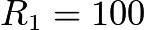$R_1 = 100$ inches,$R_2 = 60$ inches, and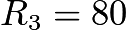$R_3 = 80$ inches, respectively. The ball always remains in contact with the track and does not slip. What is the distance the center of the ball travels over the course from A to B?$[asy] pair A,B; size(8cm); A=(0,0); B=(480,0); draw((0,0)--(480,0),linetype($

[Om’s solution]  First, we need to find the total distance of the track. The circumference of a semicircle is radius times pi, so the circumference of the three semicircles is their radi times pi added together. That is 100pi + 60pi + 80pi, which equals 240pi. We are not done yet, because the question asks us how far the center of the ball goes. The diameter of the ball is 4, so the distance from the track to the center of the ball is the radius, or 2. This means we can subtract 2pi from all the downward parts, since the ball is inside those parts, and add 2pi to all the upward parts, since the ball is above those parts and adds the lenght of its radius to that semicircle. If you add 2pi and then subtract 2pi twice from 240pi, you get A, or 238pi.

Week of Oct 16 – Oct 22: The AMC8 test is 4 weeks away.   Let’s do a few timed practices.  Starting this week, the weekly practice will involve doing one previous test in a 40-minute sitting.  Remember for AMC8 there is no calculator, no team work, no web help, and also no penalty for GUESSING. I will also ask you to submit your solutions to three of the harder problems at the end of each test. This will give you a chance to work on the harder problems if you don’t get to finish the entire test in 40 minutes.  The best solution(s) will be posted and acknowledged.

Click GoogleForm to submit solutions to the 3 written problems by Oct 22.  The answers key to the 2014 AMC8 test can be found at  http://artofproblemsolving.com/wiki/index.php?title=2014_AMC_8_Answer_Key.

Oct 16: AMC8 test 2014, https://www.artofproblemsolving.com/wiki/index.php?title=2014_AMC_8_Problems.  Please take the test before looking at the three problems below.

Oct 17-18:  (Problem 22) A 2-digit number is such that the product of the digits plus the sum of the digits is equal to the number. What is the units digit of the number?

[Stephen’s solution] Let x be the 10s digit and y be the units digit.  xy+x+y=10x+y,  xy=9x, y=9.  Therefore, 9 is the answer.

Oct 19-20: (Problem 23) Three members of the Euclid Middle School girls’ softball team had the following conversation.
Ashley: I just realized that our uniform numbers are all 2-digit primes.
Bethany: And the sum of your two uniform numbers is the date of my birthday earlier this month.
Caitlin: That’s funny. The sum of your two uniform numbers is the date of my birthday later this month.
Ashley: And the sum of your two uniform numbers is today’s date.

What number does Caitlin wear?

[Allen’s solution] http://imgur.com/ECmpoH0.

[Gyan’s solution]  Caitlin wears the number 11.  Since a month has 31 days, the only possible 2-digit primes two of which add up to less than 31 are 11, 13, and 17, one for each player. From the clues that are given to us we can determine that (Note: A = Ashley’s number, B = Bethany’s number, and C = Caitlin’s number) A + B > B + C > A + C, which implies B > A > C.  Therefore, we make A = 13, B = 17, and C = 11.

Oct 21-22: (Problem 24) One day the Beverage Barn sold 252 cans of soda to 100 customers, and every customer bought at least one can of soda. What is the maximum possible median number of cans of soda bought per customer on that day?

[Allen’s solution]

[Gyan’s solution] The maximum possible median of cans is 3.5. Since there are 100 people, the median is the average of the 50th and 51st customers if they are ordered in the least to greatest number of cans they bought. To make the maximum median we make the first 49 costumers buy 1 can. Then 252 – 49 = 203 cans are left to divide up among 51 customers. 203/51 = 3 50/51 so the 50th person has 3 cans and the rest have 4. The average of 3 and 4 is 3.5 so the maximum median number of cans is 3.5.

Week of Oct 9 – Oct 15: This week we continue with inequalities.  Remember to flip the direction of your inequality sign if you divide or multiply by a negative number.Some of the problems come from Art of Problem Solving.

Answers: (-6,1), 57, 10, 3, 8, 23, 222

Oct 9: What interval consists of all$w$ that satisfy neither$-2(6+2w)\le -16$ nor$-3w\ge 18$ ?

Oct 10: How many integers$n$ satisfy the inequality$-8\pi\le n\le10\pi$?

Oct 11What is the sum of the positive integers that are solutions of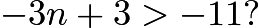$-3n +3 >-11?$

Oct 12: How many integral solutions exist for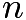$n$ if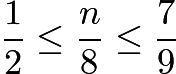$\frac12\le\frac n8\le\frac79$?

Oct 13: What integer$n$ has the property that$5^{96}$ is greater than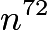$n^{72}$ and$5^{96}$ is less than$(n+1)^{72}$ ?

Oct 14: What is the largest integer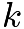$k$ such that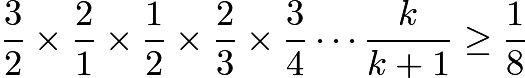$\frac{3}{2}\times\frac{2}{1}\times\frac{1}{2}\times\frac{2}{3}\times\frac{3}{4}\cdots\frac{k}{k+1}\ge\frac{1}{8}$  ?

Oct 15: Find the number of positive integers$n$ that are solutions to the simultaneous system of inequalities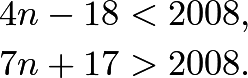Happy October!

Week of Oct 2 – Oct 8: This week we embark on something new, inequalities.  The first few problems are “routine” practice and the later ones are more interesting.  Remember to flip the direction of your inequality sign if you divide or multiply by a negative number.Some of the problems come from Intro to Algebra, Art of Problem Solving.

Answers: (-infinity, 2), (-infinity,7], (-infinity, -12), no solution, (5, infinity), (-1,4), [-2,1], 0, 9918, 12, 1007

Oct 2: Solve the following inequalities.  In your answer please use an interval to represent a range.  For example, for$x \le 2$ please write (-infinity, 2]; for$-3 < x \le 3$, please write (-3,3]; for$x>2$ or$x\le 0$, please write (-infinity, 0) or (2,infinity).

1.$2x-3 < 5-2x$
2.$7x-4(x+4)\le 12-x$

Oct 3: Solve the following inequalities.

1.$7-{3\over 4} x > 16$
2.$2+x > 5-3x > 8$

Oct 4Solve the following inequalities.

1.$4-3x+7 < 5x+19 < 8x+4$
2.$4-3x+7 < 5x+19 < 4x+23$

Oct 5: What is the length of the interval of solutions to the following inequalities?

1.$-4\le 3x+2\le 5$
2.$2y -4\le y+7 < 3y-15$

Oct 6:  How many positive integers n satisfy the following relationship?$9 < \sqrt{n} < 100$

Oct 7: How many integers n satisfy the following relationship?$9 < n^2 < 100$

Oct 8: How many positive integers n satisfy the following relationship?${1\over 3} < {n\over {n+1}} < {2016\over 2018}$